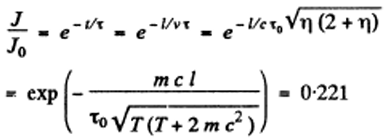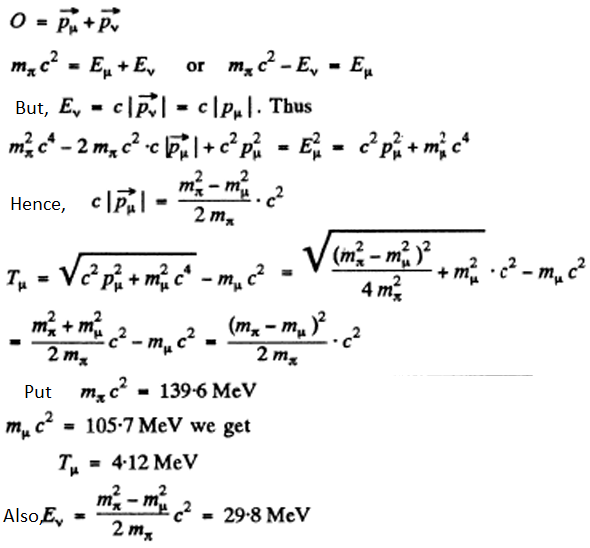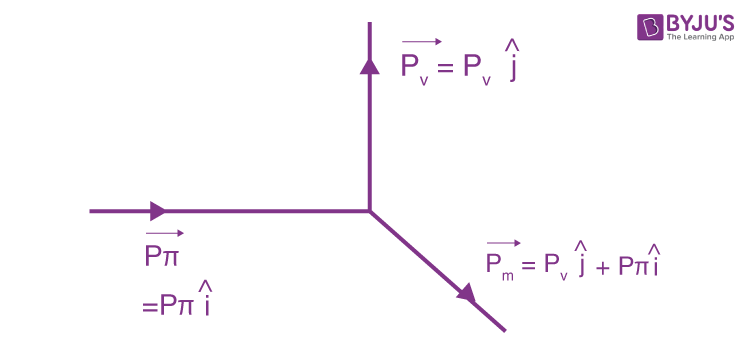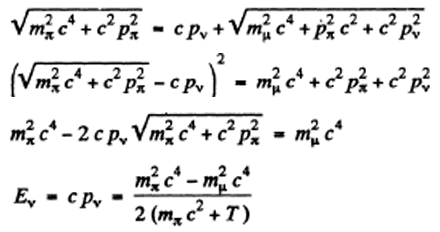# I.E. Irodov Solutions on Elementary Particles

I.E. Irodov Solutions Chapter 6.7 Elementary Particles is a premier study tool for students who are looking to develop a fresh approach in solving questions given in this chapter. Elementary particles, also known as fundamental particles, are subatomic particles which cannot be broken down into further sub-structures. Students can find detailed answers provided in the I.E. Irodov solutions which will further help them to take their preparation to a higher level and understand this chapter in a better way.

### I.E. Irodov Solutions on Elementary Particles

1: Calculate the kinetic energies of protons whose momenta are 0.10, 1.0, and 10 GeV/c, where c is the velocity of light.

Solution:

1. $T = \sqrt{c^2p^2+m_o^2c^4}-m_oc^2$

Thus, T = 5.3 MeV for p = 0.10 GeV/c = 5.3 x 10-3 GeV

T = 0.433 GeV for p = 1.0 GeV/c

T = 9.106 GeV for p = 10 GeV/c

Here we have used mo c2 = 0.938 GeV

2: Find the mean path travelled by pions whose kinetic energy exceeds their rest energy η = 1.2 times. The mean lifetime of very slow pions is ζo = 25.5 ns.

Solution:

1. Energy of pions is (1 + η) mo c2 and is equal to (mo c2)/ √(1-β2)

Hence 1/ √(1-β2) = 1 + η of pion. Hence time dilation factor is 1 + √ and the distance traversed by the pion in its lifetime will be

c β 𝜏0/ √(1-β2) = c 𝜏o √(η(2+ η)) = 15.0 metres

On substituting the values of various quantities. (Note. The factor 1/√(1-β2) can be looked at as a time dilation effect in the laboratory frame or as length contraction factor brought to the other side in the proper frame of the pion).

3: Negative pions with kinetic energy T = 100 MeV travel an average distance l = 11 m from their origin to decay. Find the proper lifetime of these pions.

Solution:

1. From the previous problem, l = c 𝜏o √(η(2+ η))

where η = (T/m π c2), mx is the rest mass of pions.

Substitution gives 𝜏o = l/c√(η(2+ η)) = 2.63 ns = lmxc/√T(T+2m π c2)

where we have used η = 0.716

4: There is a narrow beam of negative pions with kinetic energy T equal to the rest energy of these particles. Find the ratio of fluxes at the sections of the beam separated by a distance l = 20 m. The proper mean lifetime of these pions is ζo = 25.5 ns.

Solution:

1. Here η = T/mc2 = 1, so the life time of the pion in the laboratory frame is η = (1+ η) 𝜏o =2 𝜏o

The law of radioactive decay implies that the flux decreases by the factor.5: A stationary positive pion disintegrated into a muon and a neutrino. Find the kinetic energy of the muon and the energy of the neutrino.

Solution:

1. Energy-momentum conservation implies6: A stationary positive muon disintegrated into a positron and two neutrinos. Find the greatest possible kinetic energy of the positron.

Solution:

1. Solution: The reaction is,

μ+ ->e+ + ve+vμ

The neutrinoes are massless. The positron will carry largest momentum if both neutriones (ve and vμ) move in the same direction in the rest frame of the nuon. Then the final product is effectively a two body system is

(Te)max = [(mμ – mc)2]/2mμ ] c2

On substituting values, we get (Te)max =52.35 MeV

7: A stationary neutral particle disintegrated into a proton with kinetic energy T = 5.3 MeV and a negative pion. Find the mass of that particle. What is its name?

Solution:

1. By conservation of energy-momentum

Mc2 = Ep + Ex

$O = \vec{P_p} + \vec{P_\pi}$

Then, $m^2_\pi c^4 = E_\pi^2 - \vec{P_\pi}c^2 = (Mc^2-E_p)^2-c^2 \vec{P_p}$

=M2c4 – 2Mc2Ep + mp2c4

This is a quadratic equation in M, M2 – 2(Ep/c2)M + mp2 – mπ2 = 0

Using Ep = mpc2 + T and solving we get

$(M -\frac{E_p}{c^2})^2= \frac{E_p^2}{c^4}-m_p^2+m_\pi^2$

Hence,$M = \frac{E_p}{c^2} + \sqrt{\frac{E_p^2}{c^4}-m_p^2+m_\pi^2}$

on putting values, we get M = 1115.4 MeV/c2

From the table of masses we identify the particle as a ∧ particle.

8: A negative pion with kinetic energy T = 50 MeV disintegrated during its flight into a muon and a neutrino. Find the energy of the neutrino outgoing at right angles to the pion's motion direction.

Solution:

1. By conservation of energyWhere Ev = energy of the neutrino.

on writing $\sqrt{m_\pi^2 c^4 + c^2p^2_\pi}=m_\pi c^2+T$

on putting values, we get Ev = 21.93 MeV.

9: A positron with kinetic energy T = 750 keV strikes a stationary free electron. As a result of annihilation, two gamma quanta with equal energies appear. Find the angle of divergence between them.

Solution:

1. By momentum conservation

$\sqrt{E^2-m_e^2c^4}=2 \frac{E+m_ec^2}{2}cos(\theta/2)$

Or $cos(\theta/2) = \sqrt{\frac{E-m_ec^2}{E+m_ec^2}}=\sqrt{\frac{T}{T+2m_ec^2}}$

Or θ = 98.80.

10: Find the threshold energy of gamma quantum required to form (a) an electron-positron pair in the field of a stationary electron;

(b) a pair of pions of opposite signs in the field of a stationary proton.

Solution:

1. We know,

$E_{th}=\frac{(\sum m_i)^2-M^2}{2M}c^2$

when the projectile is a photon

(a) for γ + e- -> e- + e- + e+

Eth = [9me2-me2/2me] c2 = 2.04 MeV

(b) for γ + p -> p + π- π+

$E_{th}=\frac{(M_p+2M_\pi)^2-M^2_p}{2M_P}c^2$

=2 (mπ + m2π/Mp)c2 = 320.8 MeV

11: Protons with kinetic energy T strike a stationary hydrogen target. Find the threshold values of T for the following reactions:

(a) p+p→p+p+p+$\tilde{p}$

(b) p+p→p+p+p+πo

Solution:

1. (a) T ≥ Tth = [(16mp2-4mp2)/2mp]c2 = 5.63 GeV

(b) T ≥ Tth = [((2mp-m πo2)-4mp2)/2mp]c2 = 0.280 GeV

12: Find the strangeness S and the hypercharge Y of a neutral elementary particle whose isotopic spin projection is Tz = +1/2 and baryon charge B = +1. What particle is this?

Solution:

1. From the Gell-Mann Nishijima formula

Q=Tz + Y/2

We get, O = (1/2) + Y/2 or Y = -1

Also, Y = B+S

Or S = -2

Thus, the particle is =o0.